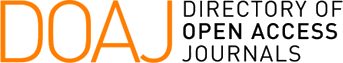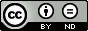### A Completely Parallelizable Algorithm for the Determinant of a Tridiagonal Matrix

A. Mahmood, D. J. Lynch, L. D. Philipp

#### Abstract

A new parallel algorithm (MIMD-PRAM class) having parallel time complexity of log2 n for computing the determinant of a tridiagonal matrix is developed. The algorithm is based on coupling the determinants of two neighboring submatrix blocks. With each coupling, the block size is increased by a factor of two until the entire determinant of an n x n matrix is found by the final coupling of two n/2 sized blocks. It is shown that the determinant of an n x n tridiagonal matrix can be computed in (3 log2 n - 2) parallel steps with a maximum parallel requirement of 7 ( n/4) + 3 processors. The algorithm achieves linear speedup as the number of processors is increased.

#### Keywords

Determinant, Tridiagonal Matrix, Parallel Algorithm, MIMD, PRAM

#### Full Text:

PDF